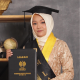Tenrycolle.com

What better to do than, share your English knowledge with other people

## What does research in the psychometric tradition typically investigate?

The psychometric tradition investigates the quantitative relationship between various classroom activities or behaviour and language achievement (Chaudron, 1988).

## What are variables, samples, and populations and why are they important in research?

As the term suggests, variables are anything that does not remain constant. In our case, it includes language proficiency, aptitude, motivation and so on (Nunan, 1992).

Populations are all individuals (students) who share one or more observable characteristics (Nunan, 1992).

Samples are a subset of individuals from a given population (Nunan, 1992).

They are essential in research because when someone conducts research, she/he collects the data from the sample; as a section of the population. Moreover, these data relate to the focused variables.

## What are the basic principles of sound experimental design?

The basic principle of sound experimental design is randomization; each individual in the population has an equal opportunity to be selected for the sample.

## What do we mean by inferential statistics?

Inferential statistics is to make inferences and predictions based on the data gathered. These will include t-test, analysis of variance (ANOVA), correlation and chi-square.

## When is it appropriate to use the following statistical procedure: t-test, analysis of variance, correlation, and chi-square?

A T-test is used when the researcher wants to compare the mean score between two groups.

Analysis of variance (ANOVA) is used when the researcher wants to compare means scores from three or more groups.

Correlation is used when the researcher wants to estimate the degree of association between two variables.

Chi-square is used when the researcher wants to calculate the difference between the expected frequencies, if the proportions were the same, and compares these with actual frequencies.

## What are the two ways of classifying variables? Elaborate your answer!

Nunan (1992) states that a variable,as the term suggests, is anything that does not remain constant. In our case, it includes language proficiency, aptitude, motivation, etc. In such a case, it is customary to distinguish between the two variables by giving them different labels. The label given to the variable that the experimenter expects to influence the other is called the independent variable. Meanwhile, the variable upon which the independent variable is acting is called the dependent variable.

Variables can also be classified according to the type of scale on which they are measured. A nominal scale measures mutually exclusive characteristics, such as sex and eye colour. An Ordinal scales are for those variables that can be given a ranking, such as first, second, or third, but the actual score itself is not given. An interval scale provides information on the ranking of scores and indicates the distance between the score. A final type of scale is the ratio scale, which measures absolute values, such as temperature.

Thus, it can be summed up that there aretwo ways of classifying variables. First, by giving different labels, namely independent variable and dependent variable. Second, according to the scale on which they are measured, namely a nominal scale, an ordinal scale, an interval scale and a ratio scale.

## What kind of situation requires an experiment as an appropriate way of gathering data?

The situation requires experiment as an appropriate way of gathering data:

The teacher has developed innovative listening material. Suppose the teacher wants to prove that this innovative listening material is significantly superior to the other traditional materials. In that case, the teacher can experiment with a classroom setting by using two groups. One which uses innovative listening materials and one which does not.

## Why is it difficult to conduct experiments in a school setting, and what do you think is the best solution?

It is difficult to conduct experiments in a school setting because it is still not rigorous enough to allow you to claim that there is a casual relationship between the independent variable (for example, innovative listening materials) and the dependent variable (the students’ test score). There is always the possibility that some factor other than the experimental materials has brought about the observed differences in the scores.

Using statistical concepts is best to experiment in a school setting. From a statistical point of view, when studying numerical data of various sorts, we will know the extent to which the data are similar and the degree to which the data differ.

## Why is the standard deviation, in addition to the mean, important in studying numerical data?

The standard deviation (SD) is important in studying numerical data because it is a measure of dispersion, giving us information on the extent to which a set of scores varies in relation to the mean (Nunan, 1992).

## What is a situation which requires analysis of variance (ANOVA) as the best technique of data analysis?

The situation requires analysis of variance (ANOVA) as the best technique of data analysis:

The researcher is interested in determining whether children’s academic performance in Math, Technology and English differed according to social-economic status and whether any effect applied equality to academic performance in both subjects. There are three groups of subjects: (1) Math, (2) English, and (3) Technology.

#### References

• Chaudron, C. 1988. Second Language Classroom – Research on Teaching and Learning. Cambridge: CUP.
• Nunan, D. 1992. Research Method in Language Learning. N.Y: CUP.##### Tenry Colle

Hi! My name is A. Tenry Lawangen Aspat Colle. I am a motivated and resourceful English educator. In addition, as the owner of @rymari.translation17 has shaped me to be a punctual and dependable translator of Indonesian to English and vice versa.